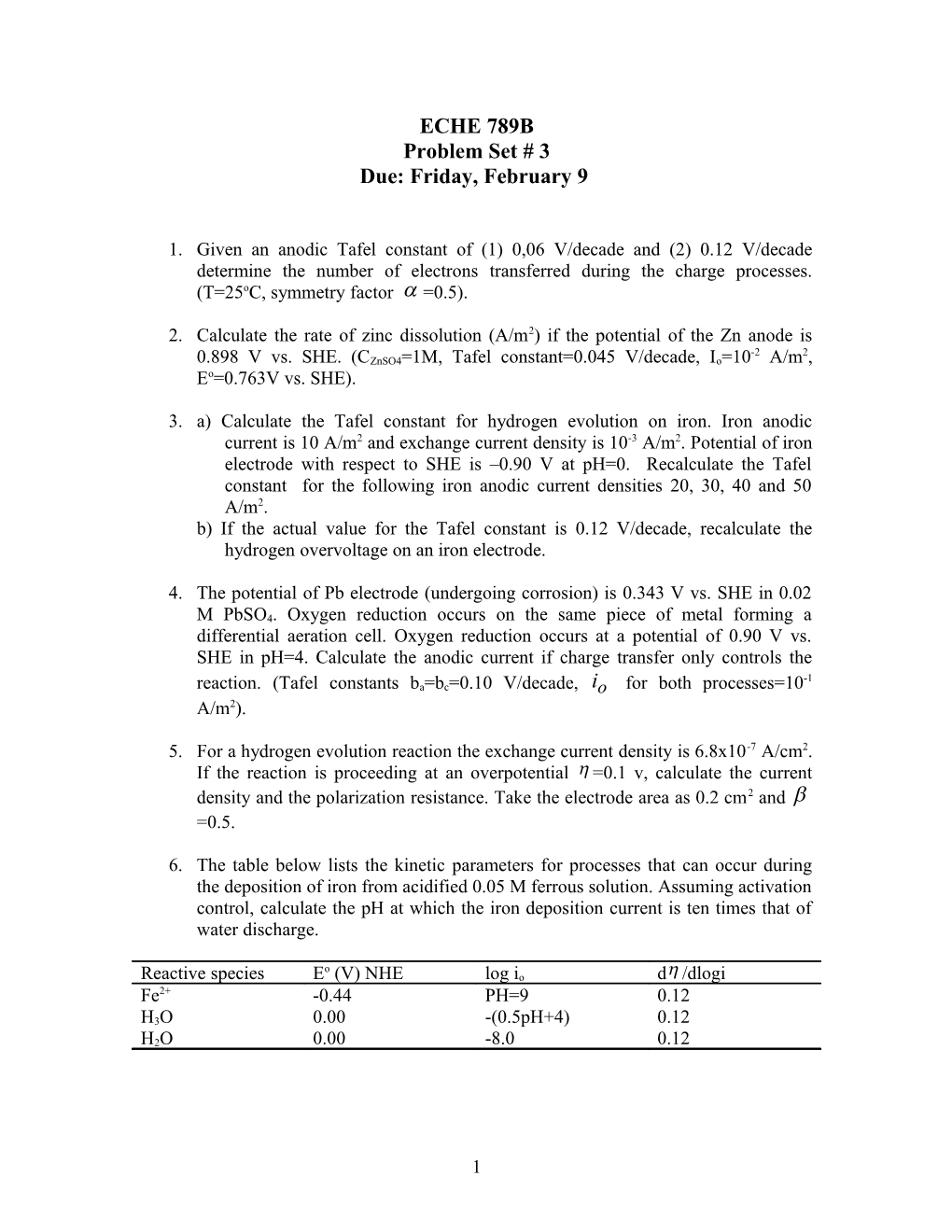# B) If the Actual Value for the Tafel Constant Is 0.12 V/Decade, Recalculate the HydrogenECHE 789B

Problem Set # 3

Due: Friday, February 9

1. Given an anodic Tafel constant of (1) 0,06 V/decade and (2) 0.12 V/decade determine the number of electrons transferred during the charge processes. (T=25oC, symmetry factor =0.5).
1. Calculate the rate of zinc dissolution (A/m2) if the potential of the Zn anode is 0.898 V vs. SHE. (CZnSO4=1M, Tafel constant=0.045 V/decade, Io=10-2 A/m2, Eo=0.763V vs. SHE).
1. a) Calculate the Tafel constant for hydrogen evolution on iron. Iron anodic current is 10 A/m2 and exchange current density is 10-3 A/m2. Potential of iron electrode with respect to SHE is –0.90 V at pH=0. Recalculate the Tafel constant for the following iron anodic current densities 20, 30, 40 and 50 A/m2.

b) If the actual value for the Tafel constant is 0.12 V/decade, recalculate the hydrogen overvoltage on an iron electrode.

1. The potential of Pb electrode (undergoing corrosion) is 0.343 V vs. SHE in 0.02 M PbSO4. Oxygen reduction occurs on the same piece of metal forming a differential aeration cell. Oxygen reduction occurs at a potential of 0.90 V vs. SHE in pH=4. Calculate the anodic current if charge transfer only controls the reaction. (Tafel constants ba=bc=0.10 V/decade, for both processes=10-1 A/m2).
1. For a hydrogen evolution reaction the exchange current density is 6.8x10-7 A/cm2. If the reaction is proceeding at an overpotential =0.1 v, calculate the current density and the polarization resistance. Take the electrode area as 0.2 cm2 and =0.5.
1. The table below lists the kinetic parameters for processes that can occur during the deposition of iron from acidified 0.05 M ferrous solution. Assuming activation control, calculate the pH at which the iron deposition current is ten times that of water discharge.

Reactive species / Eo (V) NHE / log io / d/dlogi
Fe2+ / -0.44 / PH=9 / 0.12
H3O / 0.00 / -(0.5pH+4) / 0.12
H2O / 0.00 / -8.0 / 0.12

### Construction of Evan’s Diagrams

1. Construct an E-log(i) Evan’s Diagram for the corrosion of tin in hydrogen saturated oxygen free solution in which activity of Sn is 0.4 (molar scale). The data for the cathodic reaction are as follows:

## Given:

ac=1.24

bc=0.11

Assume:

a)charge transfer kinetics

b)high field approximation applies

c)ba=0.1

d)ioa=10-10 mA/cm2

Calculate:

a)corrosion current

b)exchange current density for hydrogen evolution

c)protection current to prevent corrosion for PH2=1 atm.

The corrosion potential for Sn is –0.08V. If the hydrogen evolution reaction is limited by the diffusion of H+ species to the metal sites, reconstruct the Evan’s diagram for this case.

1. Using the following data, construct an E-log(i) Evan’s Diagram for the corrosion of nickel in saturated oxygen solution of Ni-0.23 (molar scale).

Assume:

a)charge transfer kinetics

b)high field approximation applies

Given:

a)corrosion potential=0.1V

b)exchange current density for nickel dissolution 10-5 A/cm2.

c)Anodic ba=0.12

d)Cathodic bc=-0.1

e)Cathodic intercept a=-0.6

Calculate:

a)corrosion current

b)exchange current density for oxygen on nickel dissolution 10-5 A/cm2

c)the protection current to prevent corrosion for PO2= 1 atm.

d)pH of the solution if the partial pressure of oxygen is 0.5 atm; 1 atm; 2 atm and 3 atm.

1. Using the following data, construct an E-log(i) Evan’s Diagram for the corrosion of copper in saturated oxygen solution of Cu=10-6M (molar scale) in 1.0 M NaOH

Assume:

a)charge transfer kinetics

b)high field approximation applies

Given:

c)exchange current density for copper dissolution 10-9 A/cm2.

d)exchange current density for oxygen reduction 10-8 A/cm2.

e)Anodic ba=0.1

f)Cathodic bc=-0.12

g)Cathodic intercept a=-0.7

Calculate:

a)corrosion current

b)Ecorr

c)the protection current to prevent corrosion for PO2= 1 atm.

1. Using the following data, construct an E-log(i) Evan’s Diagram for the corrosion of cobalt in saturated oxygen solution of Co-0.3 (molar scale).

Assume:

a)charge transfer kinetics

b)high field approximation applies

Given:

c)corrosion potential=0.2V

d)exchange current density for nickel dissolution 10-7 A/cm2.

e)anodic ba=0.12

f)Cathodic bc=-0.1

g)Cathodic intercept a=-0.8

Calculate:

a)corrosion current

b)exchange current density for oxygen on nickel dissolution 10-5 A/cm2

c)pH of the solution if the partial pressure of oxygen is: 0.5 atm: 1 atm; 2 atm and 3 atm.

d)the protection current to prevent corrosion for PO2= 1 atm.

5. Using the following data, construct an E-log(i) Evan’s Diagram for the corrosion of iron in saturated oxygen solution of Fe-0.3 (molar scale).

Assume:

1. charge transfer kinetics
2. high field approximation applies

Given:

c) corrosion potential=0.2V

d)exchange current density for nickel dissolution 10-7 A/cm2.

e) ba=0.12

f)Cathodic bc=-0.1

g)Cathodic intercept a=-0.7

Calculate:

a)corrosion current

b)exchange current density for oxygen on nickel dissolution 10-5 A/cm2

c)pH of the solution if the partial pressure of oxygen is: 0.5 atm: 1 atm; 2 atm and 3 atm.

d)the protection current to prevent corrosion for PO2= 1 atm.

6. Using the following data, construct an E-log(i) Evan’s Diagram for the corrosion of silver in 0.3 M HCl:

Assume:

a)charge transfer kinetics

b)high field approximation applies

Given:

a)ioH2=5x10-7 Am-2

b)ioAg=5x10-6 Am-2

c)bc=-0.09 V

d)ba=-0.12 V

e)EoH+/H2=0.0

f)EoAg+/Ag=0.799

g)[Ag+]=10-26

Calculate:

a)corrosion current

b)corrosion potential

c)the protection current to prevent corrosion

7. Using the following data construct an E-log(i) Evan’s Diagram for the corrosion of zinc on hydrogen saturated oxygen free solution of pH=0.8 and Zn2+=0.15 M (molar scale).

Assume:

a)charge transfer kinetics

b)high field approximation applies

Given:

a)ioH2=3x10-11 Am-2

b)anodic ()=1.24V

c)c=-0.118 V

d)corrosion potential=-0.34V

e)EoH+/H2=0.0

f)EoZn2+/Zn=0.799=-0.763 V

Calculate:

a)corrosion current

b)exchange current density

c)protection current required to reduce corrosion current to zero

d)the corrosion rate in mpy

8. Using the following data construct an E-log(i) Evan’s Diagram for the corrosion of cadmium on hydrogen saturated oxygen free solution of pH=4.0 and Cd2+=0.1 M(molar scale).

Assume:

a)charge transfer kinetics

b)high field approximation applies

Given:

a)ioH2=3x10-11 Am-2

b)ba=0.1V

c)bc=-0.12V

d)cathodic intercept a=-1.4 V

e)EoH+/H2=0.0

f)EoCd2+/Cd=0.799=-0.563 V vs. SHE

Calculate:

a)corrosion current

b)exchange current density

c)protection current required to reduce corrosion current to zero

1